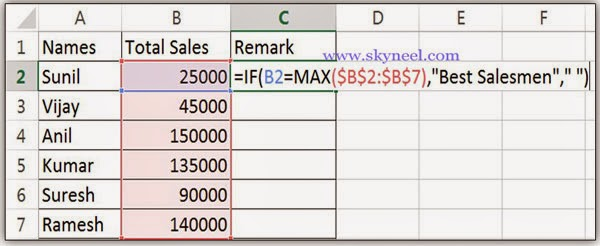# Nested Statistical If Statements with Max in Excel

Use Nested Statistical If Statements with Max in Excel. Technique used to display text or do calculation only if cell value is maximum or minimum.

How To Use Nested Statistical If Statements with Max in Excel. It is a very useful technique which is used to display text or do calculation only if cell’s value is maximum or minimum from given range of cells in active sheet. In that case of logical testing, it will contains a nested statistical function like MAX or MIN.

For example, if a salesmen total sales is maximum than all others in total sales column then print a message “Best Salesmen” in remark field. If the logical test is false, then print a blank message. When we type the formula for logical testing, it should be readable that we can use two types of cell address or references in this formula. The first reference is salesmen total sales is displays in relative cell reference while second reference represent the range of total sales of all salesmen’s in absolute cell reference.

## Nested Statistical If Statements with Max:

Max(): It’s is very important statistical function in MS Excel. This function is specially used to displays the maximum or largest value from the given range of cells.

Note: When we copy this formula in range c3:c6, the second reference of total salesmen’s sales will not be changed due to using absolute cell reference, it will be constant.

Step 1. Create the following sheet in excel.Step 2. Take cursor on cell C2 and type following formula in Remark column:  =IF (B2=MAX (B2:B6),”Best Salesmen”, ” “) then press F4 function key to convert the range B2:B6 from Relative to Absolute cell reference, it looks like given below:
=IF (B2=MAX (\$B\$2:\$B\$6),”Best Salesmen”, ” “)  , then press enter.Step 3. Now drag this formula up to cell C7 or you can also select the range from C2:C7 then press ALT+D shortcut key to view the result.I hope you like this Nested Statistical If Statements with Max guide. Give your important suggestion in the comment box and also share this article with your friends, office staff members and many more to show your knowledge in excel. Thanks.